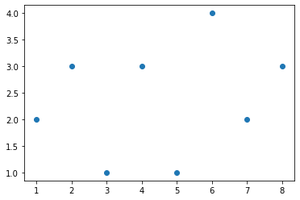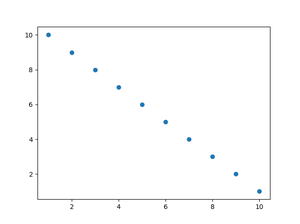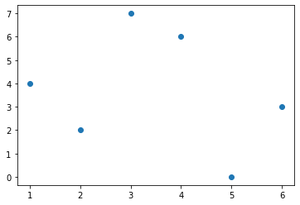GeeksforGeeks App
Open AppBrowser
Continue

# How to Plot List of X, Y Coordinates in Matplotlib?

Prerequisites:

Matplotlib is a comprehensive library for creating static, animated, and interactive visualizations in Python. To plot any data the most basic step starts with creating or loading it, this article discusses all the ways of passing the data to be plotted as list. While passing data as list one important thing to be kept in mind is to keep X,Y Lists of the same size, otherwise it will raise a ValueError.

Examples shown in this article use scatter plot, the same approaches can be applied to any other type of graph.

### Method 1: Naive method

In this method, the coordinates are simply passed as two lists.

Approach

• Import module
• Create a list for X coordinates
• Create a list for Y coordinate
• Pass these two lists to plot the function

Example:

## Python3

 `import` `matplotlib.pyplot as plt`` ` `x ``=` `[``1``, ``2``, ``3``, ``4``, ``5``, ``6``, ``7``, ``8``]``y ``=` `[``2``, ``3``, ``1``, ``3``, ``1``, ``4``, ``2``, ``3``]`` ` `plt.scatter(x, y)``plt.show()`

Output:### Method 2: Using numpy array

Function used: arrange()

Syntax: np.arange(start,end,step)

Parameters:

• Start: starting value
• End: ending value
• Step: step size, by default it is 1.

Approach

• Import module
• Create numpy array for coordinates
• Pass these arrays to plot

Example:

## Python3

 `# importing Matplotlib and Numpy Packages``import` `numpy as np``import` `matplotlib.pyplot as plt`` ` `# generating two arrays from 10 to 1 and from 1 to 10``x ``=` `np.arange(``1``, ``11``, ``1``)  ``y ``=` `np.arange(``10``, ``0``, ``-``1``)  `` ` `# plot our list in X,Y coordinates``plt.scatter(x, y)``plt.show()`

Output:### Method 3: List of lists

Creating lists of list of all points to be plotted can also one of the ways of achieving our requirement. After creating such a list it cannot be directly passed to plot, first a transpose needs to taken to get x and y coordinates.

Approach

• Import module
• Create list of lists for coordinates
• Take transpose
• Pass the coordinates to the plot

Example

## Python3

 `# importing Matplotlib and Numpy Packages``import` `numpy as np``import` `matplotlib.pyplot as plt`` ` `# The data are given as list of lists (2d list)``data ``=` `np.array([``    ``[``1``, ``4``],``    ``[``2``, ``2``],``    ``[``3``, ``7``],``    ``[``4``, ``6``],``    ``[``5``, ``0``],``    ``[``6``, ``3``]``])``# Taking transpose``x, y ``=` `data.T`` ` ` ` `# plot our list in X,Y coordinates``plt.scatter(x, y)``plt.show()`

Output:My Personal Notes arrow_drop_up